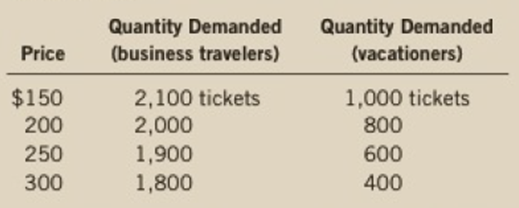Chapter 5, Problem 2PA

Chapter
Section
Textbook Problem

Suppose that business travelers and vacationers have the following demand for airline tickets from New York to Boston:a. As the price of tickets rises from $200 to$250, what is the price elasticity of demand for (i) business travelers and (ii) vacationers? (Use the midpoint method in your calculations.)b. Why might vacationers have a different elasticity from business travelers?

Subpart (a):

To determine
The calculating price elasticity of demand.

Explanation

1. (i) By midpoint method; the price elasticity of demand for business travelers takes place when the price of tickets rises from $200 to$250 is calculated as follows:

Price elasticity of demandBusiness travellers=(20001900)((2000+1900)2)÷(250200)((250+200)2)=1001950÷50225=1001950×22550=0.23

The price elasticity of demand for business travelers is 0

Subpart (b):

To determine
Relevance of price elasticity of demand.

Still sussing out bartleby?

Check out a sample textbook solution.

See a sample solution

The Solution to Your Study Problems

Bartleby provides explanations to thousands of textbook problems written by our experts, many with advanced degrees!

Get Started

List and describe six costs of inflation.

Brief Principles of Macroeconomics (MindTap Course List)

VALUATION Of A CONSTANT GROWTH STOCK A stock is expected to pay a dividend of 0.50 at the end of the year (that...

Fundamentals of Financial Management, Concise Edition (with Thomson ONE - Business School Edition, 1 term (6 months) Printed Access Card) (MindTap Course List)

Why are quality costs the costs of doing things wrong?

Cornerstones of Cost Management (Cornerstones Series)